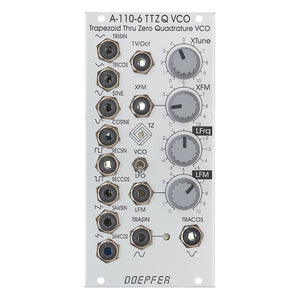# Doepfer - A-110-6: Trapezoid Thru Zero Quadrature VCO

• \$285.00

Manufacturer Description:

A-110-6 is a Trapezoid Thru Zero Quadrature VCO. The module is based on an idea by Donald Tillman from 2003 but has been revised for the use of modern electronic circuits (no OTAs/CA3280). Because of it's unique trapezoid core it's totally different compared to other VCOs. But the trapezoid core is not the only specialty: it is also a quadrature VCO and features linear thru zero frequency modulation.

The term "quadrature" means in this connection that the oscillator outputs two trapezoid waves with 90 degrees phase shift. The same as sine and cosine of a standard quadrature oscillator like the A-110-4 or A-143-9. These waveforms are called TRASIN (trapezoid sine) and TRACOS (trapezoid cosine).

The term "Thru-Zero" means that even "negative" frequencies are generated. But this a bit a misleading term as negative frequencies do not really exist. "Negative" means in this connection simply that the TRASIN/TRACOS waves will stop when the linear control voltage reaches 0V and continue with the opposite directions as the linear control voltage becomes negative and vice versa.The module has two control sections: linear and a exponential. The exponential section consists of the XTune control, the 1V/Oct input and the XFM input with the corresponding attenuator XFM. The exponential control voltage is the sum of these three voltages. The linear section consists of the LFrq control and the LFM input with the corresponding attenuator LFM. The linear control voltage is the sum of these two voltages. A dual color LED is used to display the polarity of the linear control voltage. The pitch of all outputs is determined by the control voltages of both sections. The linear section is used to control the pitch in a linear manner. When the LFrq control (LFrq means Linear Frequency Control) is fully CW the module works like a normal VCO and the LED lights red. The pitch is then controlled by the exponential section with the manual Tune control XTune and the exponential frequency control inputs 1V/Oct and XFM. 1V/Oct is used to control the pitch by a 1V/Oct CV source (e.g. sequencer or Midi/USB-to-CV interface). XFM is used to apply an exponential frequency modulation with adjustable depth (e.g. from an LFO or another VCO). As the LFrq control is turned counterclockwise starting from the fully CW position the frequency is lowered in a linear manner until the TRASIN/TRACOS waves (nearly) stop at the center position of LFrq (provided that no LFM signal is present). As the LFrq control is moved from the center towards the CCW position the waves start again but into reverse direction and the LED turns yellow. When the fully CCW position of LFrq is reached the module works again like a normal VCO. But much more exciting is the usage of the LFM input to modify the linear control voltage by an external control voltage (typically another VCO). Linear modulation by another oscillator using the thru zero feature in combination with the trapezoid waveforms generates audio spectra than cannot be obtained from any other oscillator without the thru zero function. The reason is that a "normal" VCO will simply stop as the linear control voltage becomes zero or negative. But a thru zero VCO will start again with "negative" frequencies as the the linear control voltage becomes negative.

Other waveforms like triangle, sawtooth, rectangle and sine can be obtained from the TRASIN/TRACOS signals (triangle e.g. simply by subtracting TRISIN and TRICOS, SAWSIN and SAWCOS by switching the other waveforms). All five waveforms are available as quadrature pairs (i.e. with 90 degrees phase shift):

Trapezoid (TRASIN + TRACOS)

Triangle (TRISIN + TRICOS)

Sinus (SIN + COS)

Rectangle (RECSIN + RECCOS)

Sawtooth (SAWSIN + SAWCOS)

By means

of a toggle switch the frequency range can be selected between VCO (audio range) and LFO. In LFO range the frequencies are about 1/100 compared to VCO mode.

A dual-color LED display the polarity of the linear control section.Another dual-color LED shows the signal TRASIN which is helpful in LFO mode.

Technical note regarding linear FM: The LFM CV input is DC coupled. If the input is used for linear FM in audio range and the signal applied to this input has a DC offset it will cause a small pitch shift that depends upon the value of the DC voltage. Especially when a VCA is used to change dynamically the level of the modulation signal this may generate a pitch shift caused by the control voltage feedthrough of the VCA. The control voltage feedthrough adds a DC voltage at the output which depends upon the control voltage of the VCA. For this application VCAs with a very low CV feedthough should be used or the signal output of the VCA should be AC coupled to the LFM input of the A-110-6 (e.g. by inserting a capacitor).